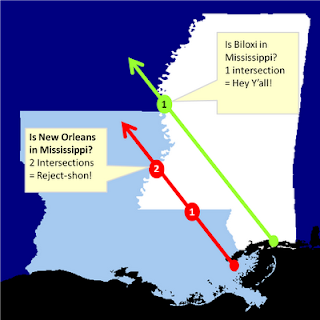## Wednesday, January 19, 2011

### Point in PolygonThe Ray Casting Method tests if a point is inside a polygon.
UPDATE: There's a newer version of this algorithm that accounts for points that fall on the boundary of a polygon which are included as inside the polygon. The title is "Point in Polygon 2: Walking the line" and was published Aug. 23, 2011.

A fundamental geospatial operation is checking to see if a point is inside a polygon.  This one operation is the atomic building block of many, many different types of spatial queries.  This operation seems deceptively simple because it's so easy to see and comprehend visually. But doing this check computationally gets quite complex.

At first glance there are dozens of algorithms addressing this challenge.  However they all have special cases where they fail.  The failures come from the infinite number of ways polygons can form which ultimately foil any sort of systematic check.  For a programmer the choice comes down to a compromise between computational efficiency (i.e. speed in this case) and thoroughness (i.e. how rare the exceptions are).

The best solution to this issue I've found is the "Ray Casting Method".  The idea is you start drawing an imaginary line from the point in question and stop drawing it when the line leaves the polygon bounding box. Along the way you count the number of times you crossed the polygon's boundary.  If the count is an odd number the point must be inside.  If it's an even number the point is outside the polygon.  So in summary, odd=in, even=out - got it?

This algorithm is fast and is accurate.  In fact, pretty much the only way you can stump it is if the point is ridiculously close to the polygon boundary where a rounding error would merge the point with the boundary.  In that case you can just blame your programming language and switch to Python.

I had no intention of implementing this algorithm myself so I googled several options, tried them out, and found a winner.  It's interesting but not surprising that most of the spatial algorithms I find and use come from computer graphics sites, usually gaming sites or computer vision sites, as opposed to geospatial sites.  My favorite ray casting point-in-polygon sample came from the "Simple Machine Forum" at "PSE Entertainment Corp".  It was posted by their anonymous webmaster.

```# Determine if a point is inside a given polygon or not
# Polygon is a list of (x,y) pairs. This function
# returns True or False.  The algorithm is called
# the "Ray Casting Method".

def point_in_poly(x,y,poly):

n = len(poly)
inside = False

p1x,p1y = poly
for i in range(n+1):
p2x,p2y = poly[i % n]
if y > min(p1y,p2y):
if y <= max(p1y,p2y):
if x <= max(p1x,p2x):
if p1y != p2y:
xints = (y-p1y)*(p2x-p1x)/(p2y-p1y)+p1x
if p1x == p2x or x <= xints:
inside = not inside
p1x,p1y = p2x,p2y

return inside

## Test

polygon = [(0,10),(10,10),(10,0),(0,0)]

point_x = 5
point_y = 5

## Call the function with the points and the polygon
print point_in_poly(point_x,point_y,polygon)
```

Easy to read, easy to use.  In a previous post on creating a dot density profile, I used the "contains" method in OGR to check randomly-generated points representing population counts against US Census Bureau tracts.  That script created a point shapefile which could then be added as a layer.  It worked great but it wasn't pure python because of OGR.  The other problem with that recipe is creating a shapefile is overkill as dot density maps are just a visualization.

I decided to build on some other posts to combine this ray casting method, PNGCanvas, and the Python Shapefile Library to create a lightweight, pure Python dot density map implementation. The following code reads in a shapefile of census tracts, looks at the population value for that tract, then randomly draws a dot within that census tract for every 50 people.  The census tract boundaries are also added to the resulting PNG image.  The conventional wisdom, especially in the geospatial world, states if you need to do a large number of costly calculations it's worth using C because Python will be much slower.  To my surprise the pure Python version was just about as quick as the OGR version.  I figured the point-in-polygon calculation would be the most costly part.  The results are close enough to warrant further detailed profiling which I'll do at some point.  But regardless this operation is much, much quicker in pure Python than I expected.

```import random
import shapefile
import pngcanvas

def pip(x,y,poly):
n = len(poly)
inside = False
p1x,p1y = poly
for i in range(n+1):
p2x,p2y = poly[i % n]
if y > min(p1y,p2y):
if y <= max(p1y,p2y):
if x <= max(p1x,p2x):
if p1y != p2y:
xints = (y-p1y)*(p2x-p1x)/(p2y-p1y)+p1x
if p1x == p2x or x <= xints:
inside = not inside
p1x,p1y = p2x,p2y
return inside

# Source shapefile - can be any polygon

# pixel to coordinate info
xdist = r.bbox - r.bbox
ydist = r.bbox - r.bbox
iwidth = 600
iheight = 500
xratio = iwidth/xdist
yratio = iheight/ydist

c = pngcanvas.PNGCanvas(iwidth,iheight,color=[255,255,255,0xff])

# background color
c.filledRectangle(0,0,iwidth,iheight)

# Pen color
c.color = [139,137,137,0xff]

# Draw the polygons
for shape in r.shapes():
pixels = []
for x,y in shape.points:
px = int(iwidth - ((r.bbox - x) * xratio))
py = int((r.bbox - y) * yratio)
pixels.append([px,py])
c.polyline(pixels)

rnum = 0
trnum = len(r.shapeRecords())
for sr in r.shapeRecords():
rnum += 1
#print rnum, " of ", trnum
density = sr.record
total = int(density / 50)
count = 0
minx, miny, maxx, maxy = sr.shape.bbox
while count < total:
x = random.uniform(minx,maxx)
y = random.uniform(miny,maxy)
if pip(x,y,sr.shape.points):
count += 1
#print " ", count, " of ", total
px = int(iwidth - ((r.bbox - x) * xratio))
py = int((r.bbox - y) * yratio)
c.point(px,py,color=[255,0,0,0xff])

f = file("density_pure.png", "wb")
f.write(c.dump())
f.close()
```

The shapefile used above can be found here.